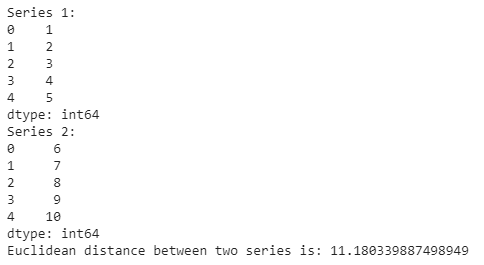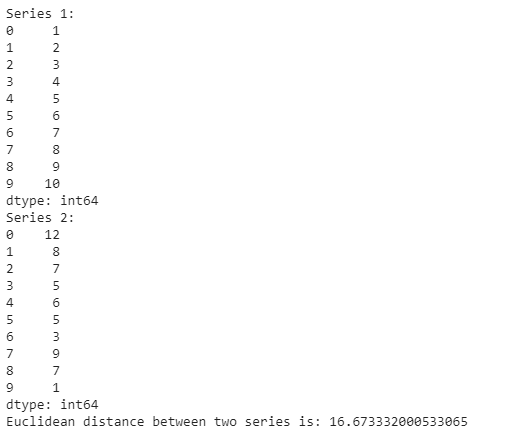Related Articles
Pandas – Compute the Euclidean distance between two series
• Last Updated : 10 Jul, 2020

There are many distance metrics that are used in various Machine Learning Algorithms. One of them is Euclidean Distance. Euclidean distance is the most used distance metric and it is simply a straight line distance between two points. Euclidean distance between points is given by the formula :We can use various methods to compute the Euclidean distance between two series. Here are a few methods for the same:
Example 1:

 import pandas as pdimport numpy as np    # create pandas seriesx = pd.Series([1, 2, 3, 4, 5])y = pd.Series([6, 7, 8, 9, 10])  # here we are computing every thing# step by stepp1 = np.sum([(a * a) for a in x])p2 = np.sum([(b * b) for b in y])  # using zip() function to create an# iterator which aggregates elements # from two or more iterablesp3 = -1 * np.sum([(2 * a*b) for (a, b) in zip(x, y)])dist = np.sqrt(np.sum(p1 + p2 + p3))  print("Series 1:", x)print("Series 2:", y)print("Euclidean distance between two series is:", dist)

Output :Example 2:

 import pandas as pdimport numpy as np    x = pd.Series([1, 2, 3, 4, 5])y = pd.Series([6, 7, 8, 9, 10])  # zip() function creates an iterator# which aggregates elements from two # or more iterablesdist = np.sqrt(np.sum([(a-b)*(a-b) for a, b in zip(x, y)]))      print("Series 1:")print(x)  print("Series 2:")print(y)  print("Euclidean distance between two series is:", dist)

Output :Example 3: In this example we are using np.linalg.norm() function which returns one of eight different matrix norms.

 import pandas as pdimport numpy as np    x = pd.Series([1, 2, 3, 4, 5])y = pd.Series([6, 7, 8, 9, 10])dist = (np.linalg.norm(x-y))  print("Series 1:")print(x)  print("Series 2:")print(y)  print("Euclidean distance between two series is:", dist)

Output :Example 4: Let’s try on a bigger series now:

 import pandas as pdimport numpy as np    x = pd.Series([1, 2, 3, 4, 5, 6, 7, 8, 9, 10])y = pd.Series([12, 8, 7, 5, 6, 5, 3, 9, 7, 1])dist = np.sqrt(np.sum([(a-b)*(a-b) for a, b in zip(x, y)]))  print("Series 1:")print(x)  print("Series 2:")print(y)  print("Euclidean distance between two series is:", dist)

Output :Attention geek! Strengthen your foundations with the Python Programming Foundation Course and learn the basics.

To begin with, your interview preparations Enhance your Data Structures concepts with the Python DS Course. And to begin with your Machine Learning Journey, join the Machine Learning – Basic Level Course

My Personal Notes arrow_drop_up